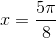## Example Questions

### Example Question #66 : Trigonometry

If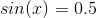, what is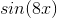ifis betweenand?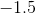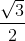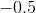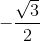Explanation:

Recall that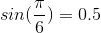.

Therefore, we are looking for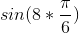or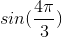.

Now, this has a reference angle of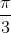, but it is in the third quadrant. This means that the value will be negative. The value of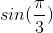is. However, given the quadrant of our angle, it will be.

### Example Question #67 : Trigonometry

What is the sine of the angle formed between the origin and the point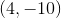if that angle is formed with one side of the angle beginning on the-axis and then rotating counter-clockwise to?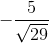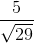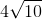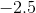Explanation:

You can begin by imagining a little triangle in the fourth quadrant to find your reference angle.  It would look like this: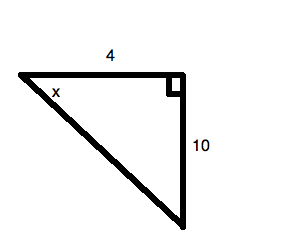Now, to find the sine of that angle, you will need to find the hypotenuse of the triangle. Using the Pythagorean Theorem,, whereandare leg lengths andis the length of the hypotenuse, the hypotenuse is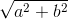, or, for our data: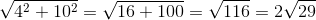The sine of an angle is: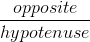For our data, this is: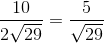Since this is in the fourth quadrant, it is negative, because sine is negative in that quadrant.

### Example Question #1 : How To Find Negative Sine

What is the sine of the angle formed between the origin and the point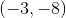if that angle is formed with one side of the angle beginning on the-axis and then rotating counter-clockwise to?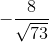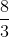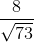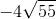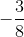Explanation:

You can begin by imagining a little triangle in the third quadrant to find your reference angle. It would look like this: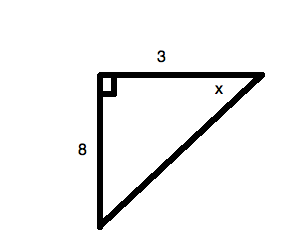Now, to find the sine of that angle, you will need to find the hypotenuse of the triangle. Using the Pythagorean Theorem,, whereandare leg lengths andis the length of the hypotenuse, the hypotenuse is, or, for our data: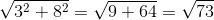The sine of an angle is:For our data, this is:Since this is in the third quadrant, it is negative, because sine is negative in that quadrant.

### Example Question #69 : Trigonometry

If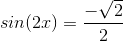, what is the value ofif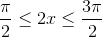?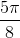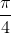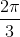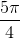Explanation:

Recall that the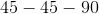triangle appears as follows in radians: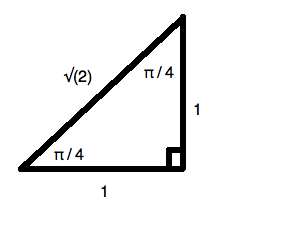Now, the sine ofis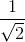. However, if you rationalize the denominator, you get: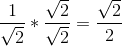Since, we know thatmust be represent an angle in the third quadrant (where the sine function is negative). Adding its reference angle to, we get: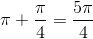Therefore, we know that: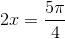, meaning that